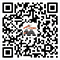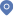首页 - 科研队伍• 研究方向：

随机（常）偏微分方程的保结构算法
• 代表作：

```1. (with T. Dang and J. Hong) Weak intermittency of stochastic heat equation under discretizations. J. Differential Equations 333 (2022), 268–301.

2. (with J. Hong and D. Sheng) Influence of numerical discretizations on hitting probabilities for linear stochastic parabolic systems. J. Complexity 70 (2022), Paper No. 101634, 29 pp.

3. A symplectic discontinuous Galerkin full discretization for stochastic Maxwell equations. SIAM J. Numer. Anal. 59 (2021), no. 4, 2197–2217.

4. (with J. Hong, D. Jin and L. Sun) Asymptotically-preserving large deviations principles by stochastic symplectic methods for a linear stochastic oscillator. SIAM J. Numer. Anal. 59 (2021), no. 1, 32–59.

5. (with J. Hong) Symplectic Runge-Kutta semidiscretization for stochastic Schrödinger equation. SIAM J. Numer. Anal. 54 (2016), no. 4, 2569–2593.```
• 联系方式：

办公室：中科院数学院科技综合楼320房间 电　话：0086-10-82541033 邮　箱：chenchuchu@lsec.cc.ac.cn